# Aldehydes and Ketones – Organic Chemistry – list of solved problems

Go to the page with the solutions to the problems.

## Aldehydes and Ketones – problem list

### Problem 1)

Identify the product obtained in each of the following reactions: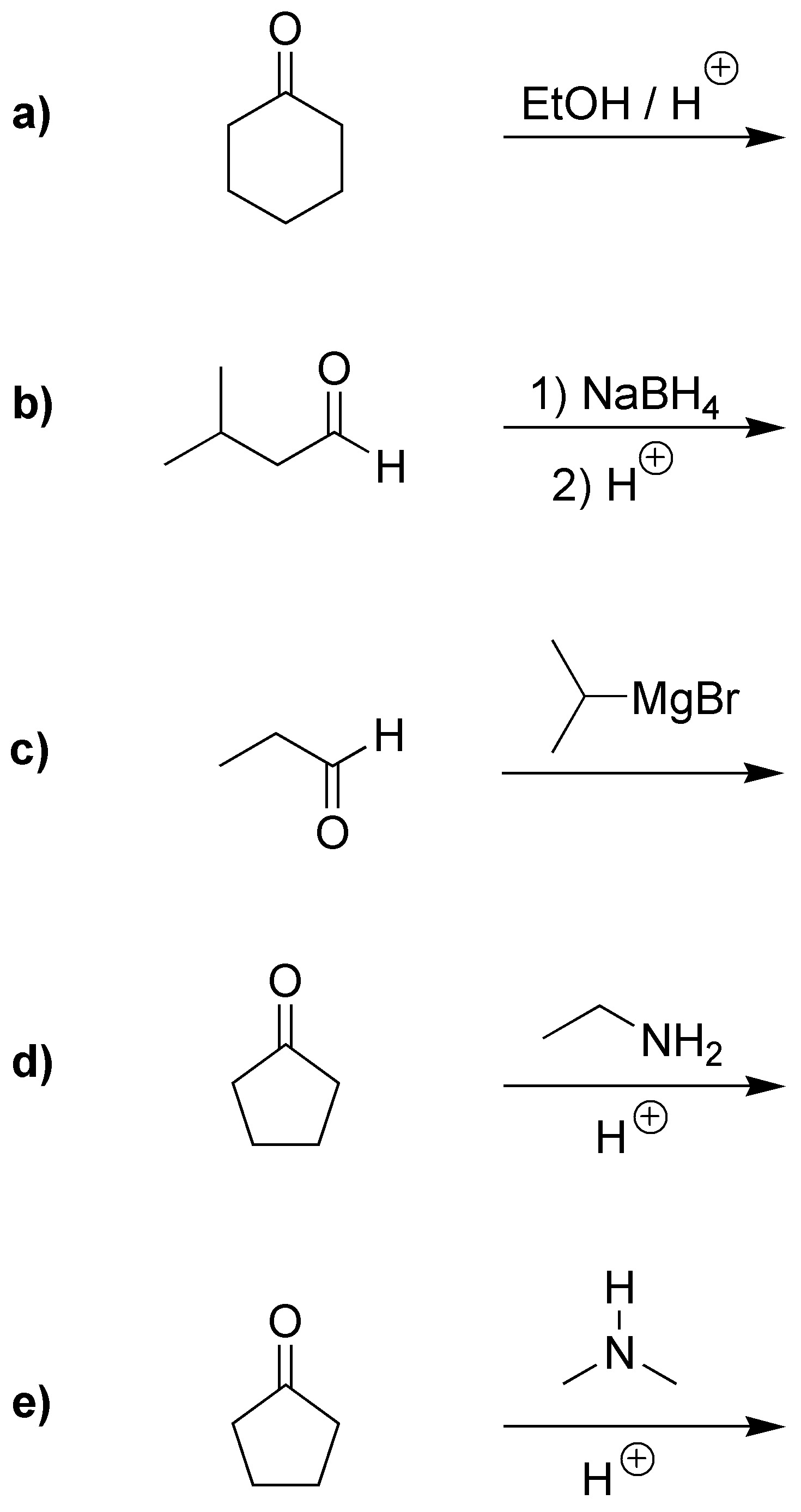### Problem 2)

Indicate the reagent needed to carry out the following transformations: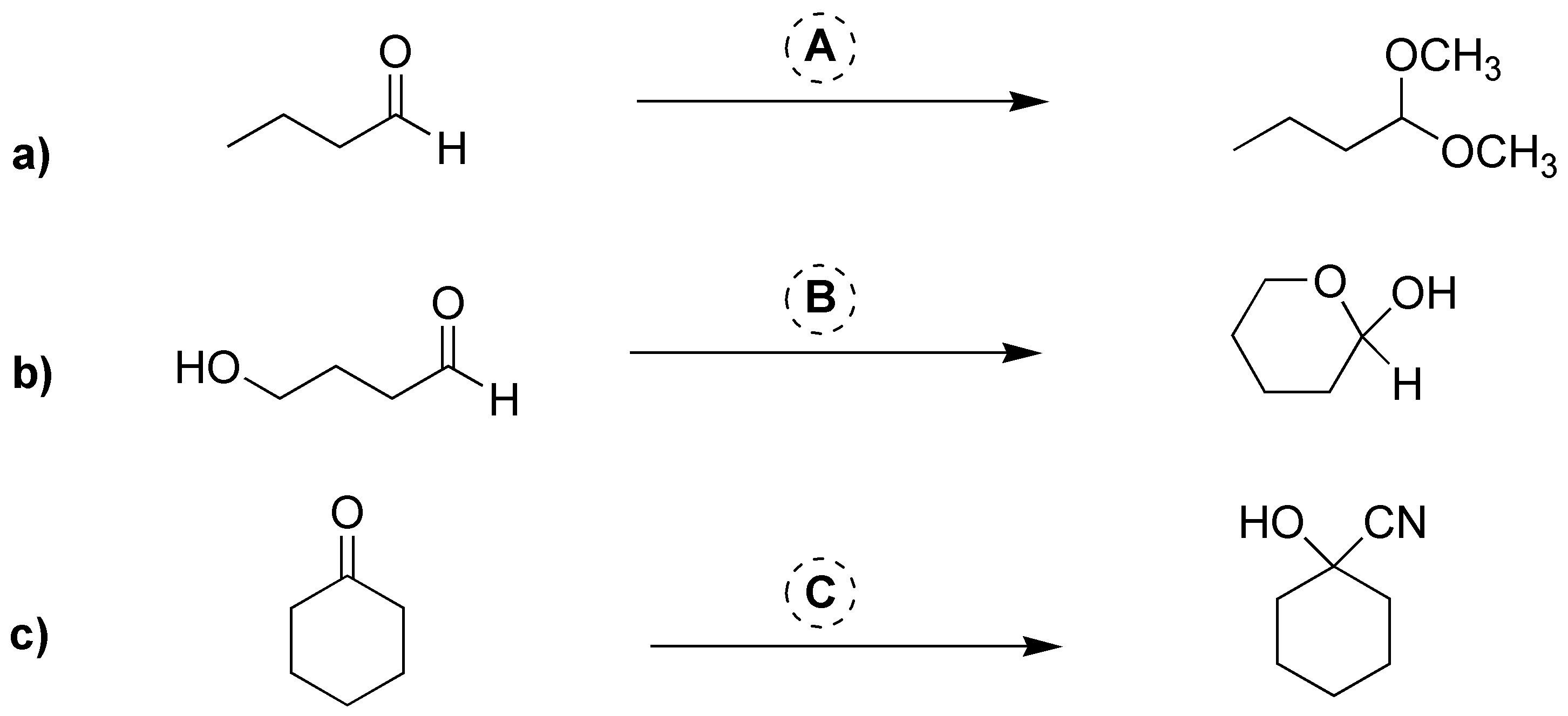### Problem 3)

Complete the following reactions: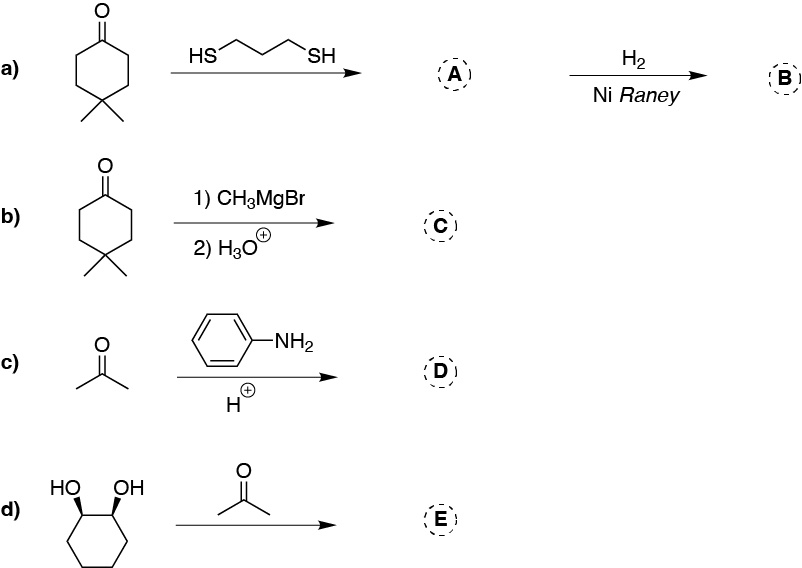### Problem 4)

Choose the appropriate carbonyl compound to obtain the following products: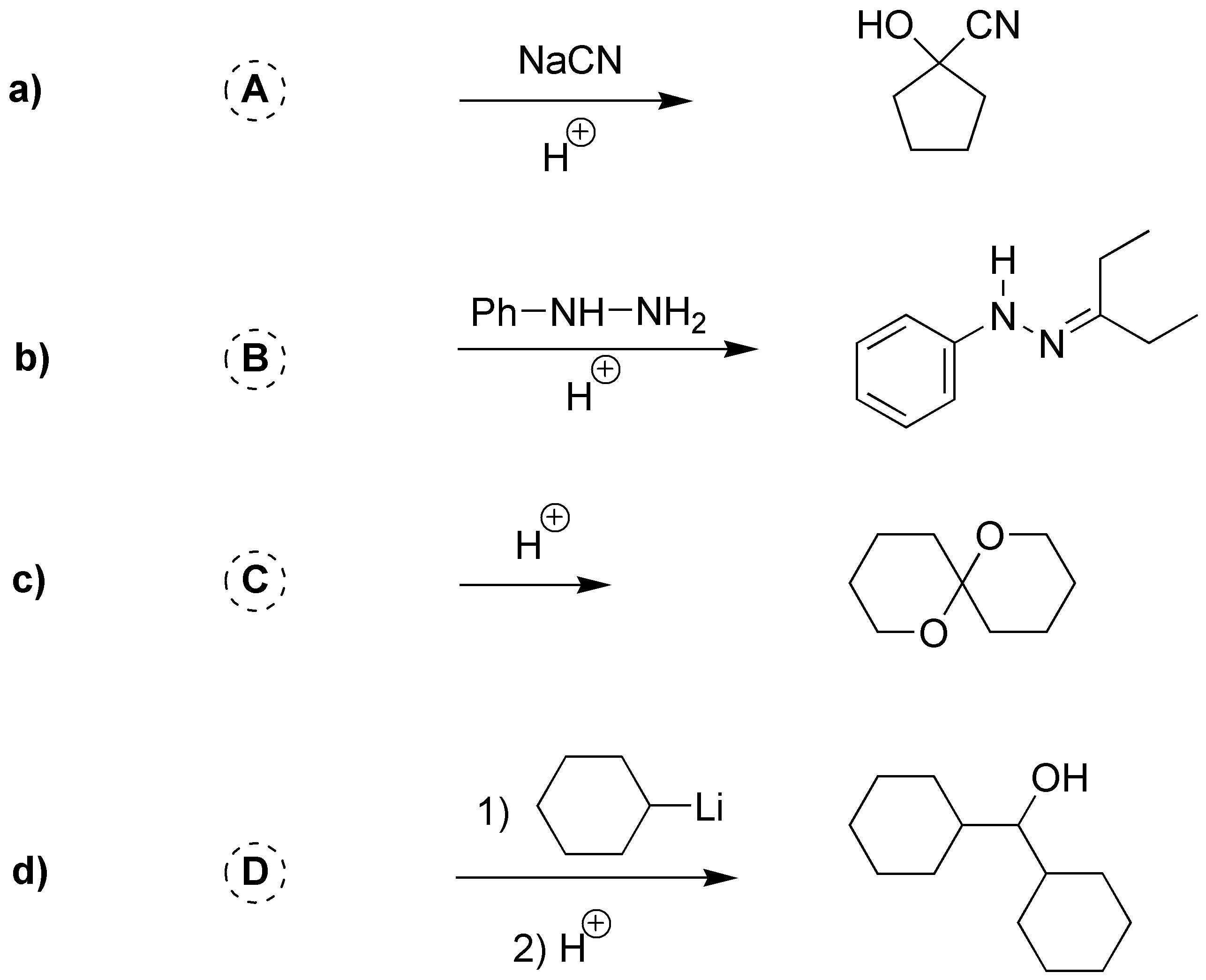### Problem 5)

What products are obtained in the following oxidation reactions of aldehydes and ketones?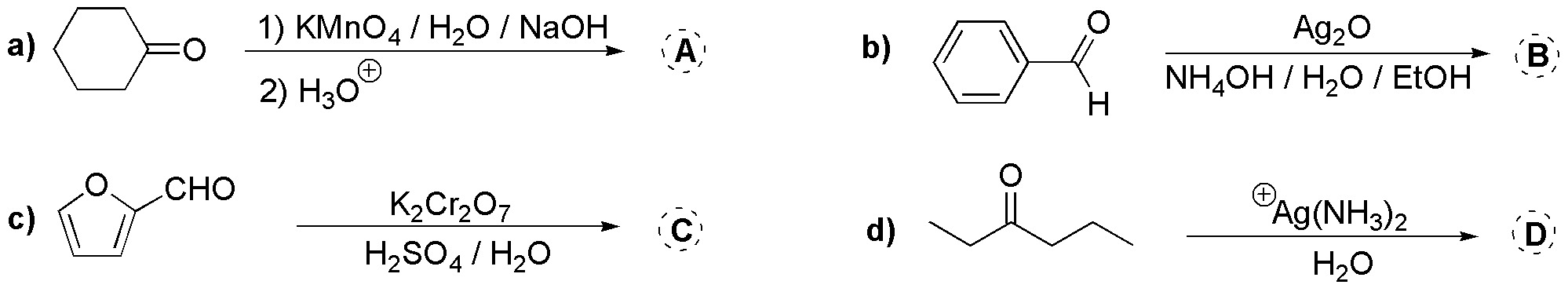### Problem 6)

Predict the major product in the reaction of phenylmethylketone (acetophenone), describing the intermediate formed, if we use as oxidizing agent the carboxylic peracetic acid MCPBA.

### Problem 7)

Write the product(s) obtained by catalytically reducing with H2 and Pd(C) the following compounds: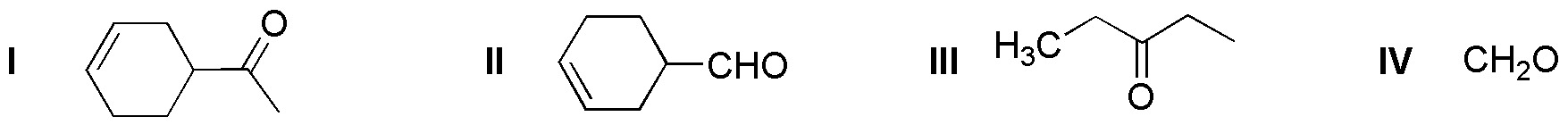### Problem 8)

Write the product obtained, from the reduction of the following compounds, in a first step with LiAlH4 in THF and subsequent acid treatment in water (H3O+).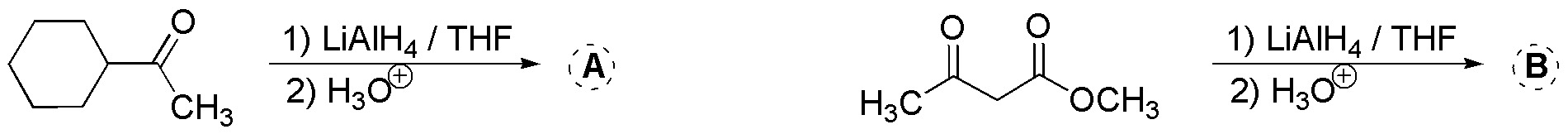### Problem 9)

Write the product obtained from the following reductions with NaBH4 in ethanol: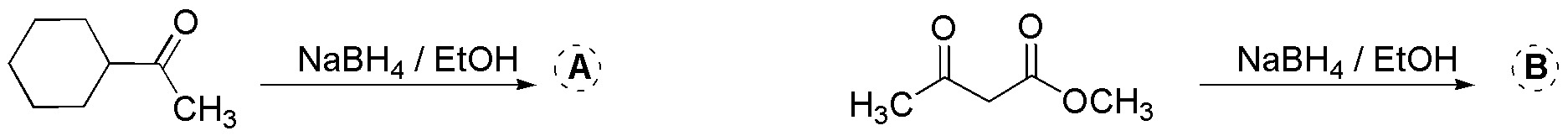### Problem 10)

What ilides and carbonyl compounds should you use in a Wittig reaction to obtain 2-methylpent-2-ene?

### Problem 11)

Write the intermediate and product obtained, from the addition with Grignard and organolithium reagents of the following ketones: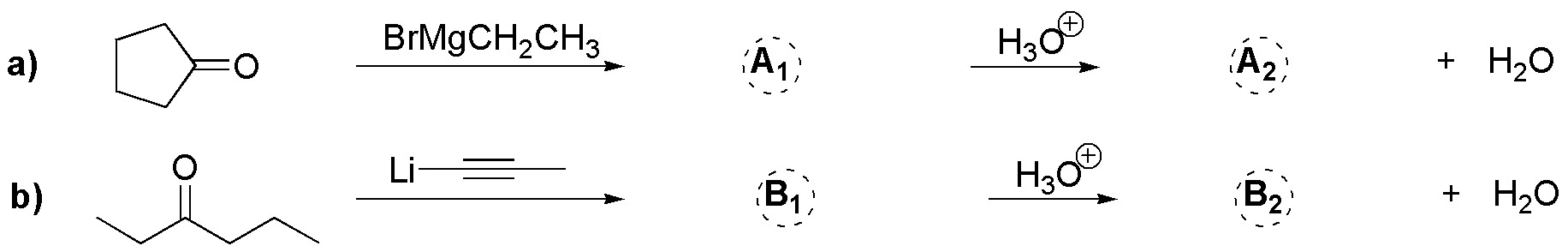### Problem 12)

Explain in a reasoned way why strongly acidic conditions, which would favor the reactivity of the carbonyl group, cannot be used in these reactions.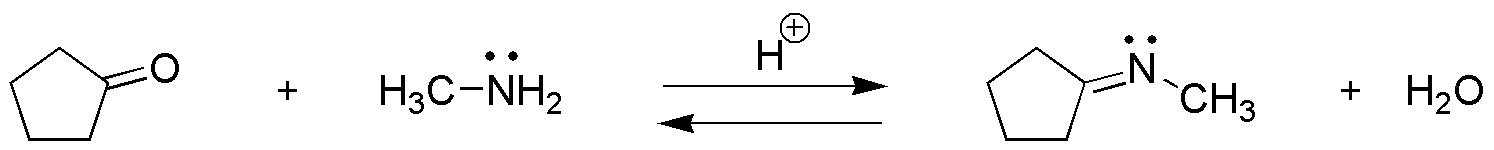### Problem 13)

Complete the following sequence of reactions: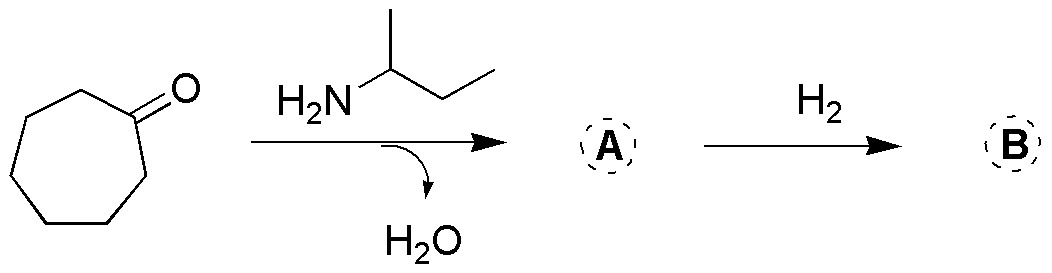### Problem 14)

Identify the compound in the following structures (I-IV), indicating a procedure for its synthesis, as well as how to regenerate the starting compound.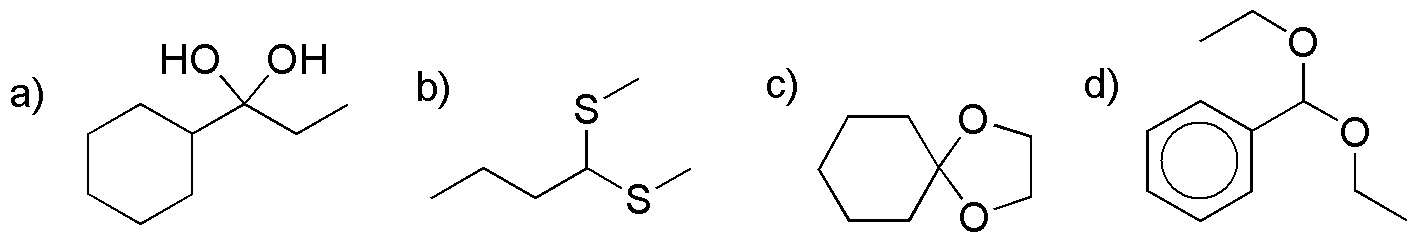### Problem 15)

Complete the following reaction scheme: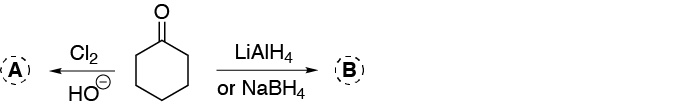### Problem 16)

Predict the major product in the reaction of the following compounds with MCPBA: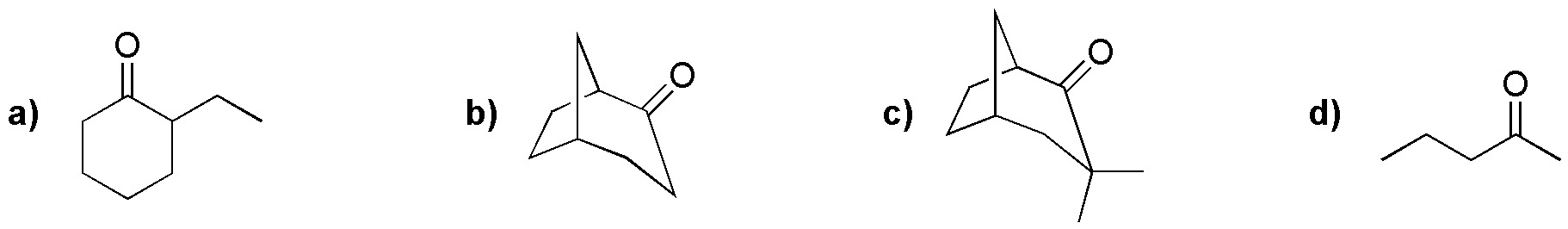### Problem 17)

Explain the formation of A from 4-hydroxypentanal when treated with 1 mol of methanol.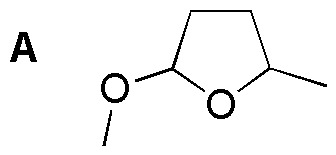### Problem 18)

Complete the following scheme: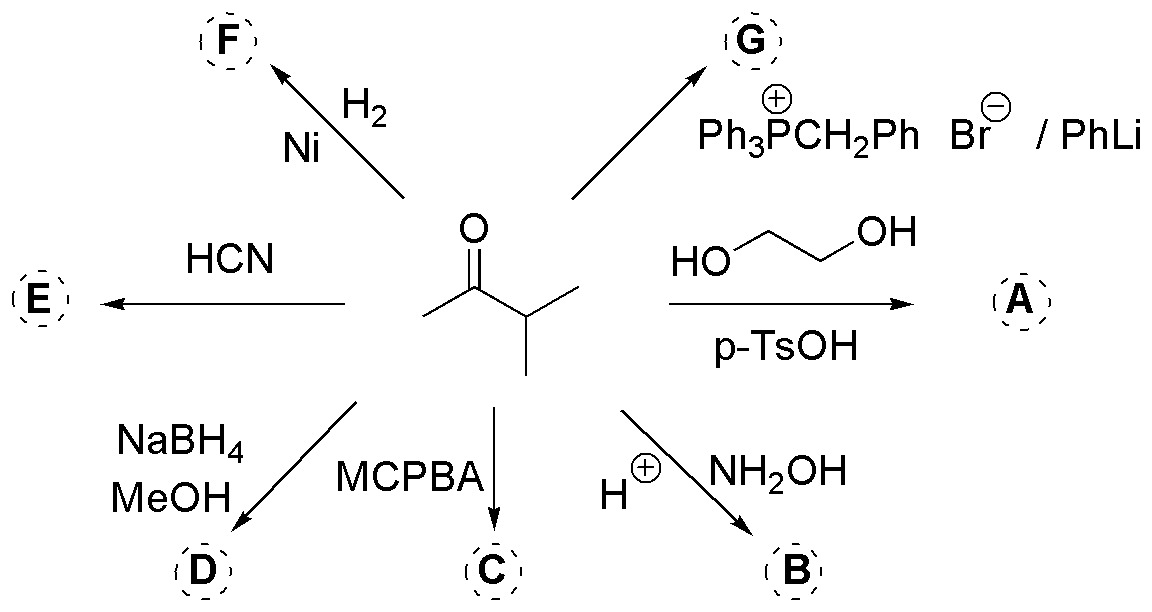### Problem 19)

Complete the following reactions: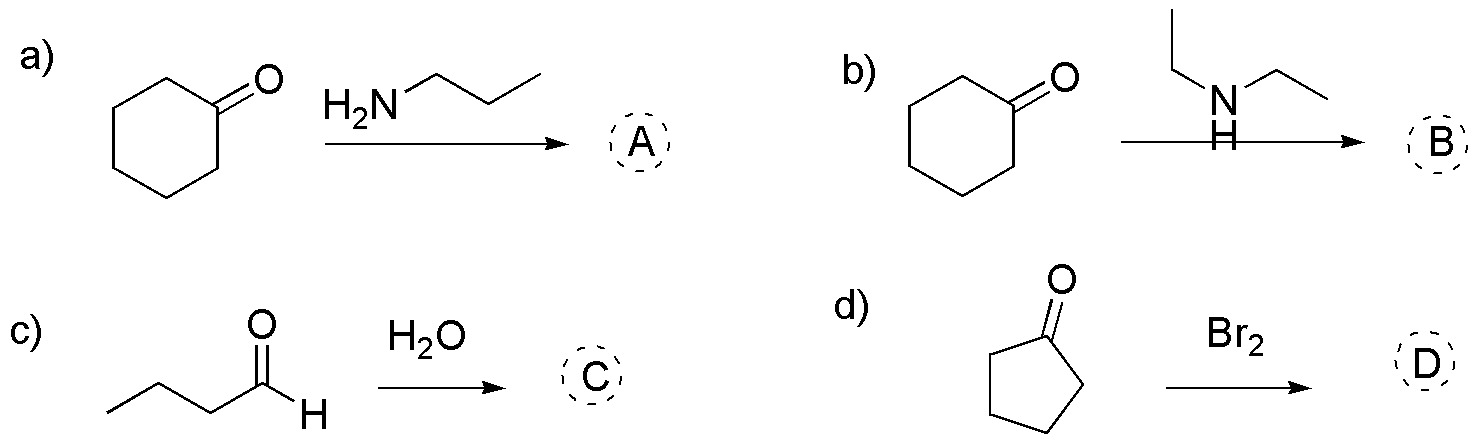### Problem 20)

Propose a procedure for the preparation of the following alcohols using cyclopentanone as the starting compound: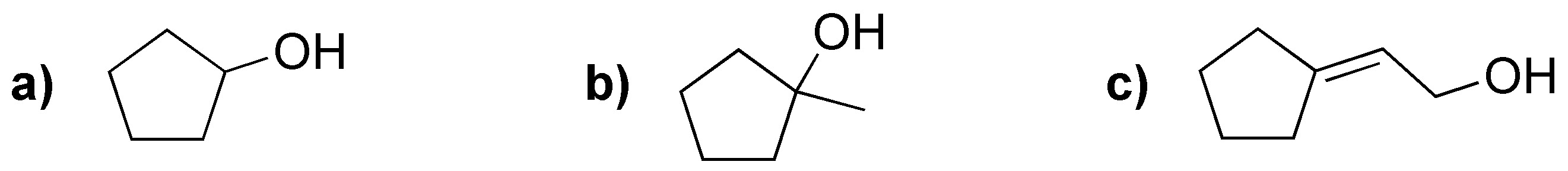### Problem 21)

Choose from the following substrates which ones would give the Cannizzaro reaction and draw the products obtained in each of them.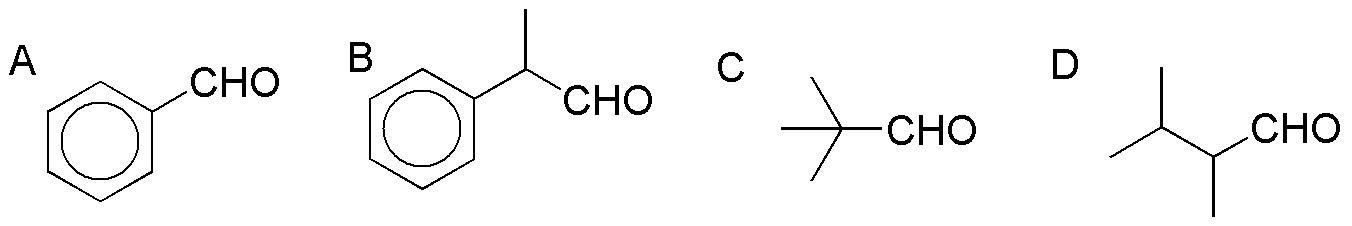### Problem 22)

Complete the following reactions (a-c):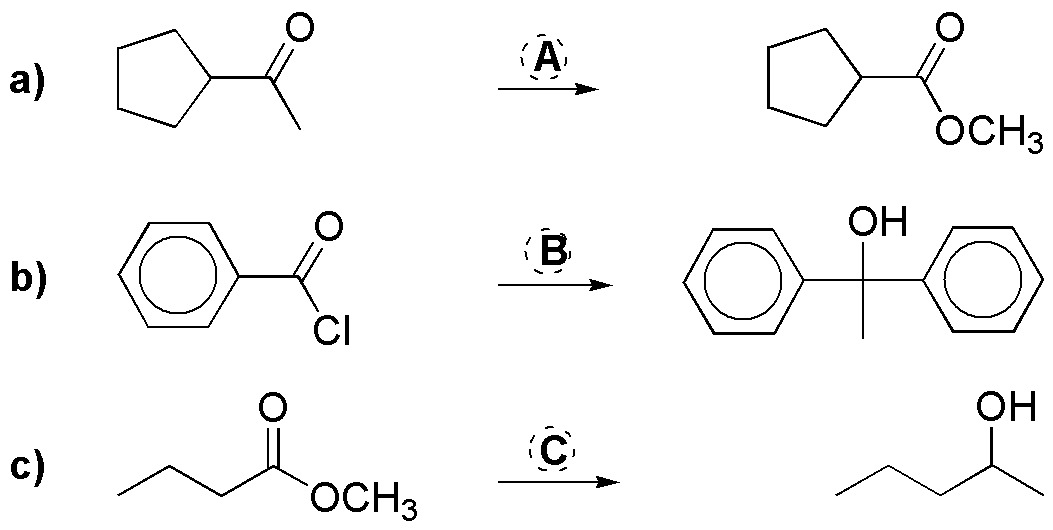### Problem 23)

Indicate the result of treating benzaldehyde with the following reactants:

• a) pentane-2,4-dione / piperidine.
• b) 1) malononitrile / triethylamine; 2) H3O+ / Δ
• c) 1) nitromethane / dimethylaminopyridine; 2) LiAlH4 / THF

### Problem 24)

Complete the following reactions (A-D):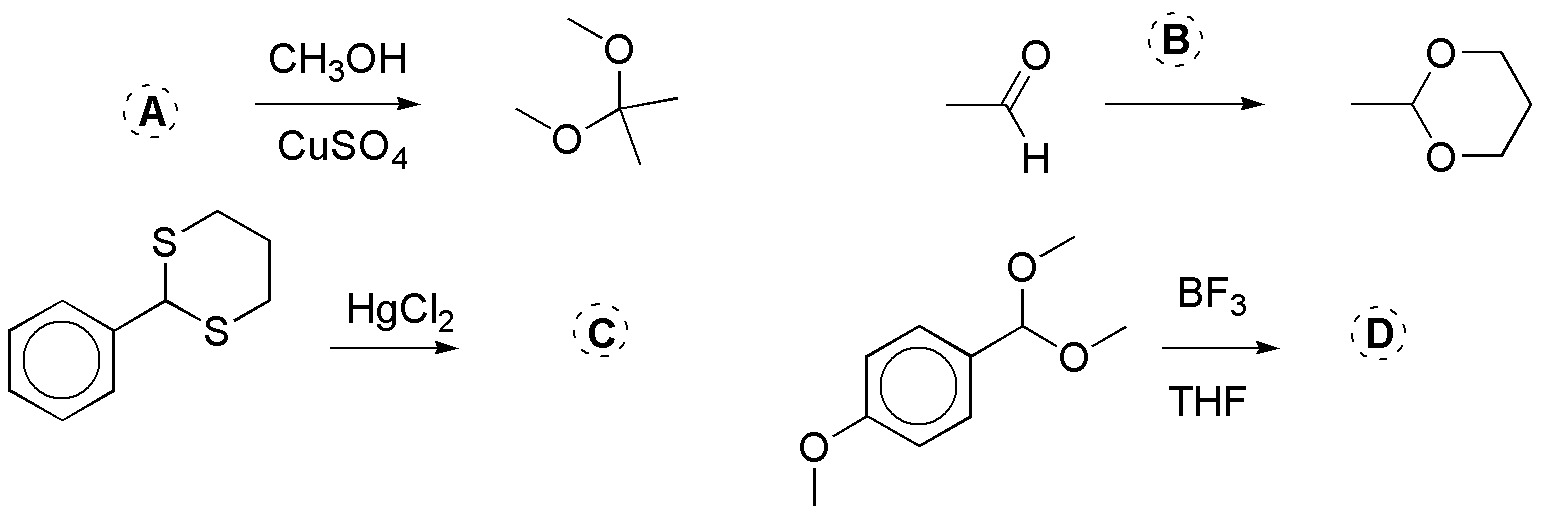Shares